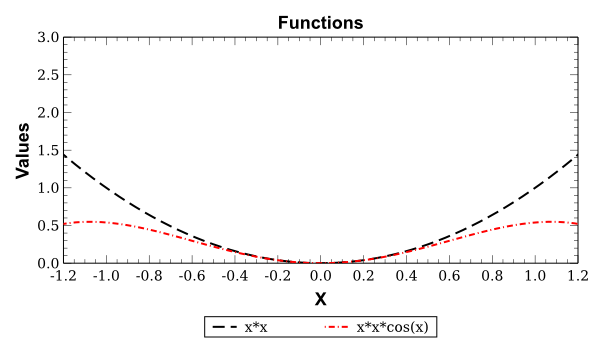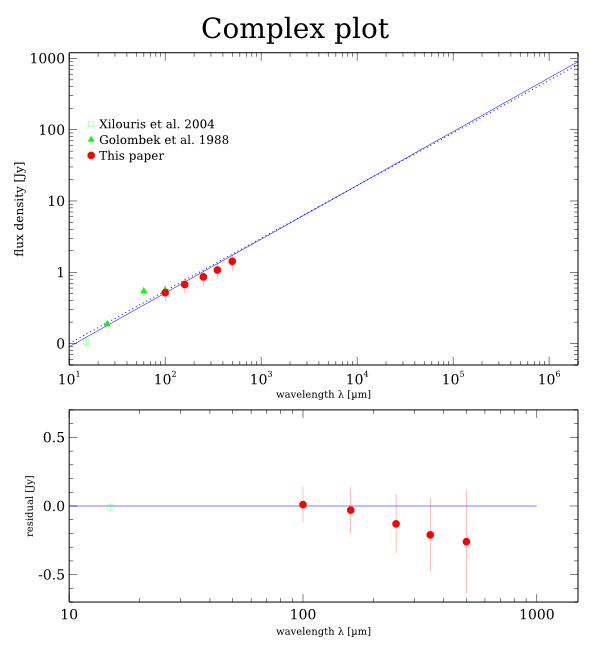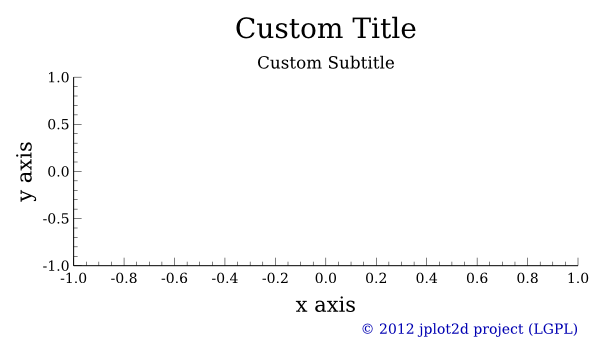# DMelt:Plots/3 Custom Plots

Limitted access. First login to DataMelt if you are a full DataMelt member. Then login to HandWiki as a user.

## Custom plots in 2D

You can create fully custom plots with arbitrary layouts by writing Jython and Java code and accessing internal methods. First, let us consider a simple plots based on the classjhplot.HPlotXY. Using the method "getPlot()" of this class one can return a plot object of thePlot class that can be changed using an arbitrary layout. Let us consider a simple example:

The output plots is:You can see thatjhplot.HPlotXY is very similar tojhplot.HPlot. However, it has several features that can help to build non-standard layouts.

In order to access all such plotting zones, you can look at the following methods:

c1=HPlotXY("Cosmology",600,700,0)
c1.visible()
ef=c1.getFactory()
plot=c1.getPlot()
env=c1.Env()

The function "getFactory()" returns class, while getPlot() returns the actual plotting area. Look at the API of these classes:

TheElementFactory class cab be used to create axis, titles, legends and subplots. In order to export plots to one of the vector-graphics formats supported by dmelt, use the usual method:

c1.export("image.svg")

(change the extension to use different file format).

Now let us build an example that shows a plot with two subplots, custom title and data points with the legends.

The output image created by the above code is:# Building titles

After you create the "plot" object of the classorg.jplot2d.element.Plot and "ef" factory object usingorg.jplot2d.element.ElementFactory class, you can add a title as:

from org.jplot2d.element import *
title = ef.createTitle("Custom Title")
title.setFontScale(2)
plot.addTitle(title)

The "title" object belongs toorg.jplot2d.element.Title class.

You can use TeX-like formatting of strings in plots. Entering math mode using a "dollar" symbol it is possible to insert Greek characters, for instance using

\\alpha - greek alpha
\\beta  - greek beta
{| class="wikitable sortable" border=1
!  # superscript symbol
|-
|}
_  # subscript symbol

Here is how to show math:

text="$A_{1.3}^{b-3/2}$"
text="$\\alpha_{1.3}^{\\beta-3/2}$"

The following special symbols are available:

• All the lower-case Greek letters.
• The following upper-case Greek letters: \Gamma, \Delta, \Theta, \Lambda, \Xi, \Pi, \Sigma, \Upsilon, \Phi, \Psi, \Omega .
• The \angstrom and \micro symbols.

To insert other symbols you can use the Unicode escape sequence \uxxxx , where xxxx is the hexadecimal code of the symbol. For example, \u2299 corresponds to the circle dot operator, which can also be used as symbol for the Sun. Use  table to look at how to map unicode characters.

# Creating Axes

To create axised for X and Y, use "createAxis" method of "ef" factory object oforg.jplot2d.element.ElementFactory class

xaxis = ef.createAxis();
yaxis = ef.createAxis();
# setting an axis transform to LOGARITHMIC
xaxis.getTickManager().getAxisTransform().setTransform(TransformType.LOGARITHMIC);

The objects "xaxis" and "yaxis" belong toAxis class. To add an axis to the plot, use these methds:

plot.addXAxis(xaxis)
plot.addYAxis(yaxis)

You may want 2 x-axes and 2 y-axes to box the plot contents. In this case, 2 x-axes or y-axes can be created and added together. The created 2 x-axes share the same tickManager. And so the 2 y-axes.

xaxes = ef.createAxes(2)
yaxes = ef.createAxes(2)
# setting an axis transform to LOGARITHMIC
xaxes.getTickManager().getAxisTransform().setTransform(TransformType.LOGARITHMIC);
plot.addYAxes(yaxes)

Let us show how to make titles, subtitles and axes in this example:

The resulting plot is:# Creating layers

A layer can associate with a x-axis and a y-axis, i.e. it is associated with a x AxisTransform and a y AxisTrasform. When adding a layer to a plot, the associated x AxisTransform and y AxisTrasform must be given as arguments, and they must already exist in the plot (by adding the x axis and y axis object).

layer = ef.createLayer();
# The xaxis and yaxis must has been added to the plot
plot.addLayer(layer, xaxis.getTickManager().getAxisTransform(), yaxis.getTickManager().getAxisTransform())

The "layer" object belongs to theorg.jplot2d.element.Layer class. You can extract layers from the plots as:

layers = plot.getLayers()

# Creating Graphs

A 2d graph can be created with 2 double array

from array import *
x = array('d',[0,1,2,3])
y = array('d',[0,2,1,4])
graph = ef.createXYGraph(x, y)
layer.addGraph(graph)  # add this graph to layer

Similarly, your code in Java will look as:

double[] x = new double[] {0,1,2,3};
double[] y = new double[] {0,2,1,4};
XYGraph graph = ef.createXYGraph(x, y);
layer.addGraph(graph)  # add this graph to layer

The "layer" object belongs to theorg.jplot2d.element.XYGraph class. You can customize the graph using the methods:

graph.setColor(Color.GREEN);
graph.setLineVisible(False);
graph.setSymbolVisible(True);
graph.setSymbolShape(SymbolShape.SQUARE);

Data points can have errors bars in X and Y. Create a XYGraph with the given x/y array, x low/high error array and y low/high error array, use this method:

graph=ef.createXYGraph(x_array, y_array, xErrorLow, xErrorHigh, yErrorLow, yErrorHigh, name)

# Creating Annotations

There are several kind of annotations can be created

1. symbol annotation A point annotation with a symbol and a text string
2. horizontal line annotation A horizontal line annotation to highlight a value.
3. vertical line annotation A vertical line annotation to highlight a value.
4. horizontal strip annotation A horizontal strip annotation to highlight a range.
5. vertical strip annotation A vertical strip annotation to highlight a range.
6. rectangle annotation A rectangle annotation to highlight a area.

The "annotations" objects belong to theorg.jplot2d.element.Annotation class. Look at how the annotations can be made:

ann0 = ef.createSymbolAnnotation(x, y, "text annotation") # symbol annotation
ann1 = ef.createHLineAnnotation(y)       #  horizontal line annotation
ann2 = ef.createHStripAnnotation(y1, y2) #  horizontal strip annotation
layer.addAnnotation(annotation) # Adding an annotation to a layer